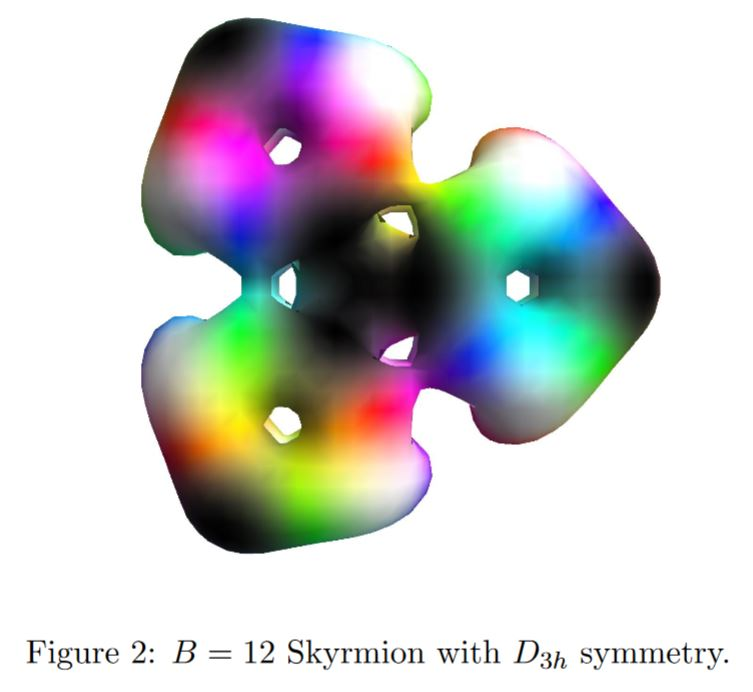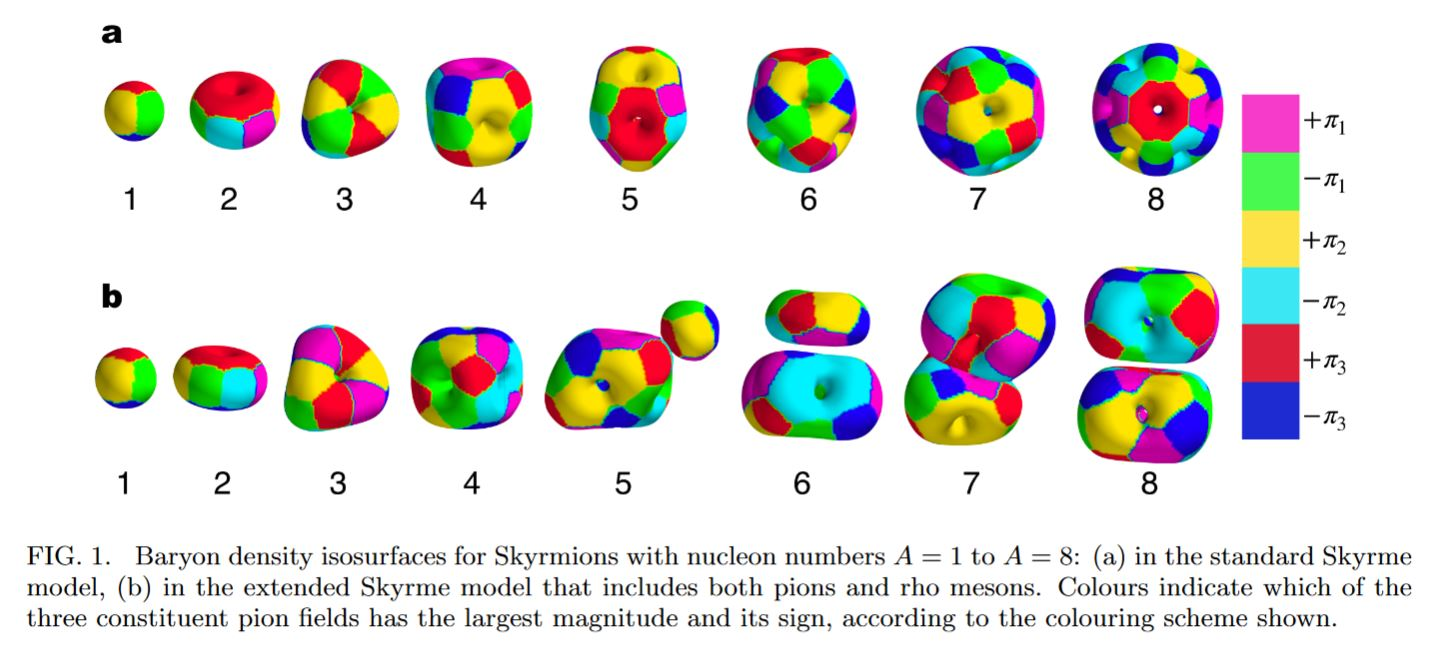# nLab nucleus (physics)

Contents

this entry is about the concept in physics. For other meanings see nucleus (disambiguation)

## Surveys, textbooks and lecture notes

#### Fields and quanta

fields and particles in particle physics

and in the standard model of particle physics:

force field gauge bosons

scalar bosons

flavors of fundamental fermions in the
standard model of particle physics:
generation of fermions1st generation2nd generation3d generation
quarks ($q$)
up-typeup quark ($u$)charm quark ($c$)top quark ($t$)
down-typedown quark ($d$)strange quark ($s$)bottom quark ($b$)
leptons
chargedelectronmuontauon
neutralelectron neutrinomuon neutrinotau neutrino
bound states:
mesonslight mesons:
pion ($u d$)
ρ-meson ($u d$)
ω-meson ($u d$)
f1-meson
a1-meson
strange-mesons:
ϕ-meson ($s \bar s$),
kaon, K*-meson ($u s$, $d s$)
eta-meson ($u u + d d + s s$)

charmed heavy mesons:
D-meson ($u c$, $d c$, $s c$)
J/ψ-meson ($c \bar c$)
bottom heavy mesons:
B-meson ($q b$)
ϒ-meson ($b \bar b$)
baryonsnucleons:
proton $(u u d)$
neutron $(u d d)$

(also: antiparticles)

effective particles

hadrons (bound states of the above quarks)

solitons

in grand unified theory

minimally extended supersymmetric standard model

superpartners

bosinos:

dark matter candidates

Exotica

auxiliary fields

# Contents

## Idea

In physics or chemistry (or quantum chemistry) an atomic nucleus – or just nucleus, for short – is a bound state of protons and neutrons. If this in turn is surrounded by a shell of electrons the result is an atom, with the nucleus at its core.

The study of nuclei is the topic of nuclear physics.

## Skyrmion models

Candidate models for baryons and even some aspects of atomic nuclei are Skyrmions (Riska 93, Battye-Manton-Sutcliffe 10, Manton 16, Naya-Sutcliffe 18).

For instance various resonances of thethe carbon nucleus are modeled well by a Skyrmion with baryon number 12 (Lau-Manton 14):graphics grabbed form Lau-Manton 14

For Skyrmion models of nuclei to match well to experiment, not just the pion field but also the heavier mesons need to be included in the construction. Including the rho meson gives good results for light nuclei (Naya-Sutcliffe 18)graphics grabbed form Naya-Sutcliffe 18

On Skyrmion models for nucleons:

Textbook account: